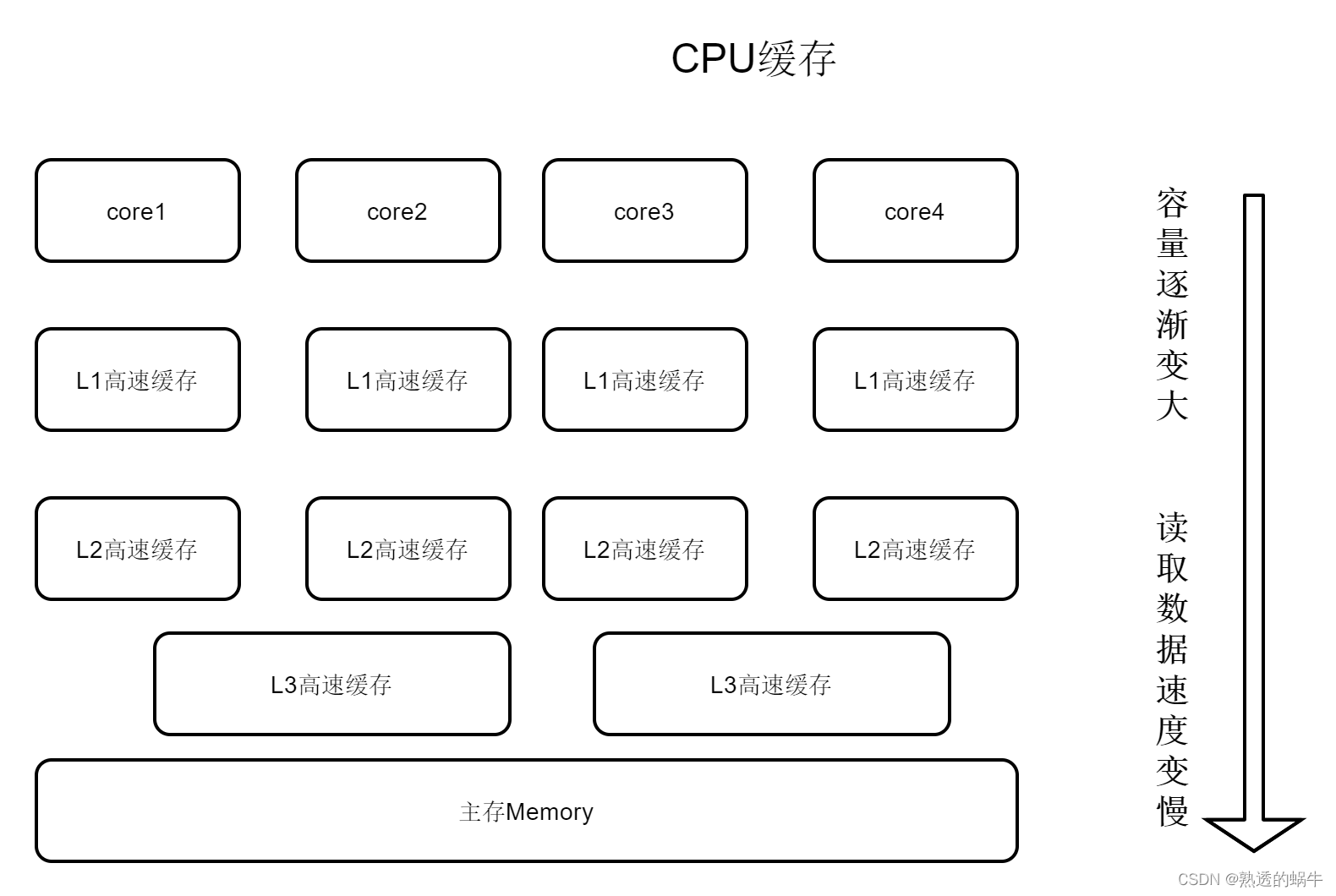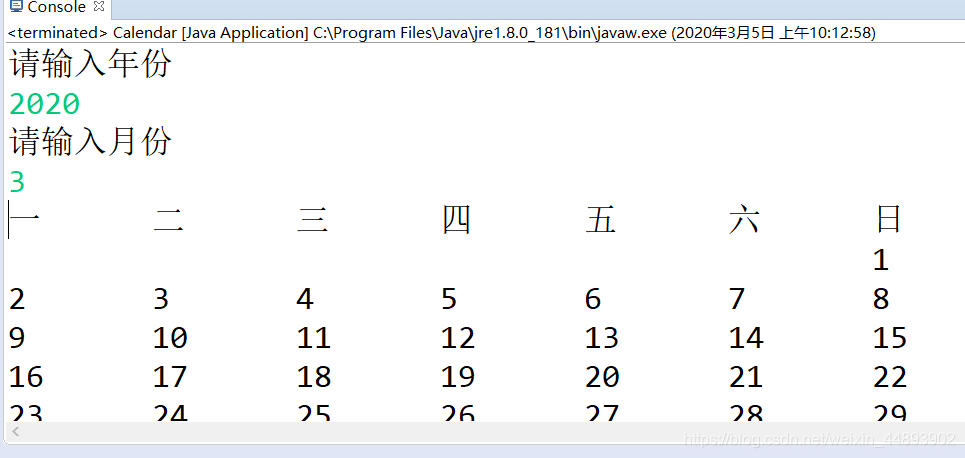+关注继续查看

# CUDA编程（五）

## 关注内存的存取模式

### 什么样的存取模式是高效的？

// __global__ 函数 (GPU上执行) 计算立方和
__global__ static void sumOfSquares(int *num, int* result, clock_t* time)
{

//计算每个线程需要完成的量
const int size = DATA_SIZE / THREAD_NUM;

int sum = 0;

int i;

//记录运算开始的时间
clock_t start;

if (tid == 0) start = clock();

for (i = tid * size; i < (tid + 1) * size; i++) {

sum += num[i] * num[i] * num[i];

}

result[tid] = sum;

if (tid == 0) *time = clock() - start;

}

thread 0 -> thread 1 -> thread 2 -> thread 3 -> thread 4 ->...### 改进存取模式

// __global__ 函数 (GPU上执行) 计算立方和
__global__ static void sumOfSquares(int *num, int* result, clock_t* time)
{

int sum = 0;

int i;

//记录运算开始的时间
clock_t start;

if (tid == 0) start = clock();

for (i = tid; i < DATA_SIZE; i += THREAD_NUM) {

sum += num[i] * num[i] * num[i];

}

result[tid] = sum;

if (tid == 0) *time = clock() - start;

}

#include <stdio.h>
#include <stdlib.h>
#include <time.h>

//CUDA RunTime API
#include <cuda_runtime.h>

//1M
#define DATA_SIZE 1048576

int data[DATA_SIZE];

//产生大量0-9之间的随机数
void GenerateNumbers(int *number, int size)
{
for (int i = 0; i < size; i++) {
number[i] = rand() % 10;
}
}

//打印设备信息
{
printf("Device Name : %s.\n", prop.name);
printf("totalGlobalMem : %d.\n", prop.totalGlobalMem);
printf("sharedMemPerBlock : %d.\n", prop.sharedMemPerBlock);
printf("regsPerBlock : %d.\n", prop.regsPerBlock);
printf("warpSize : %d.\n", prop.warpSize);
printf("memPitch : %d.\n", prop.memPitch);
printf("maxGridSize[0 - 2] : %d %d %d.\n", prop.maxGridSize, prop.maxGridSize, prop.maxGridSize);
printf("totalConstMem : %d.\n", prop.totalConstMem);
printf("major.minor : %d.%d.\n", prop.major, prop.minor);
printf("clockRate : %d.\n", prop.clockRate);
printf("textureAlignment : %d.\n", prop.textureAlignment);
printf("deviceOverlap : %d.\n", prop.deviceOverlap);
printf("multiProcessorCount : %d.\n", prop.multiProcessorCount);
}

//CUDA 初始化
bool InitCUDA()
{
int count;

//取得支持Cuda的装置的数目
cudaGetDeviceCount(&count);

if (count == 0) {
fprintf(stderr, "There is no device.\n");
return false;
}

int i;

for (i = 0; i < count; i++) {

cudaGetDeviceProperties(&prop, i);
//打印设备信息
printDeviceProp(prop);

if (cudaGetDeviceProperties(&prop, i) == cudaSuccess) {
if (prop.major >= 1) {
break;
}
}
}

if (i == count) {
fprintf(stderr, "There is no device supporting CUDA 1.x.\n");
return false;
}

cudaSetDevice(i);

return true;
}

// __global__ 函数 (GPU上执行) 计算立方和
__global__ static void sumOfSquares(int *num, int* result, clock_t* time)
{

int sum = 0;

int i;

//记录运算开始的时间
clock_t start;

if (tid == 0) start = clock();

for (i = tid; i < DATA_SIZE; i += THREAD_NUM) {

sum += num[i] * num[i] * num[i];

}

result[tid] = sum;

if (tid == 0) *time = clock() - start;

}

int main()
{

//CUDA 初始化
if (!InitCUDA()) {
return 0;
}

//生成随机数
GenerateNumbers(data, DATA_SIZE);

/*把数据复制到显卡内存中*/
int* gpudata, *result;

clock_t* time;

//cudaMalloc 取得一块显卡内存 ( 其中result用来存储计算结果，time用来存储运行时间 )
cudaMalloc((void**)&gpudata, sizeof(int)* DATA_SIZE);
cudaMalloc((void**)&time, sizeof(clock_t));

//cudaMemcpy 将产生的随机数复制到显卡内存中
//cudaMemcpyHostToDevice - 从内存复制到显卡内存
//cudaMemcpyDeviceToHost - 从显卡内存复制到内存
cudaMemcpy(gpudata, data, sizeof(int)* DATA_SIZE, cudaMemcpyHostToDevice);

// 在CUDA 中执行函数 语法：函数名称<<<block 数目, thread 数目, shared memory 大小>>>(参数...);
sumOfSquares << < 1, THREAD_NUM, 0 >> >(gpudata, result, time);

/*把结果从显示芯片复制回主内存*/

clock_t time_use;

//cudaMemcpy 将结果从显存中复制回内存
cudaMemcpy(&time_use, time, sizeof(clock_t), cudaMemcpyDeviceToHost);

//Free
cudaFree(gpudata);
cudaFree(result);
cudaFree(time);

int final_sum = 0;

for (int i = 0; i < THREAD_NUM; i++) {

final_sum += sum[i];

}

printf("GPUsum: %d  gputime: %d\n", final_sum, time_use);

final_sum = 0;

for (int i = 0; i < DATA_SIZE; i++) {

final_sum += data[i] * data[i] * data[i];

}

printf("CPUsum: %d \n", final_sum);

return 0;
}6489302/894297= 7.26


894297/ (797000 * 1000) =  0.0011221S


1M 个 32 bits 数字的数据量是 4MB。

4MB / 0.0011221S = 3564.745MB/s = 3.48GB/s


### 总结：JUC并发编程——JAVA内存模型
JUC并发编程——JAVA内存模型
21 049 023 0Java并发编程的艺术(十三)——锁优化

866 0Java并发编程的艺术(二)——重排序

668 01. 在线程中执行任务 1.1 串行的执行任务 这是最经典的一个最简单的Socket server的例子，服务器的资源利用率非常低，因为单线程在等待I/O操作完成时，CPU处于空闲状态。
1185 0[C++][编程风格]优质代码的十条建议
1096 0《Java并发编程的艺术》一一2.4　本章小结
1139 0《Java并发编程的艺术》一一3.2　重排序
1474 0720 0
77

0